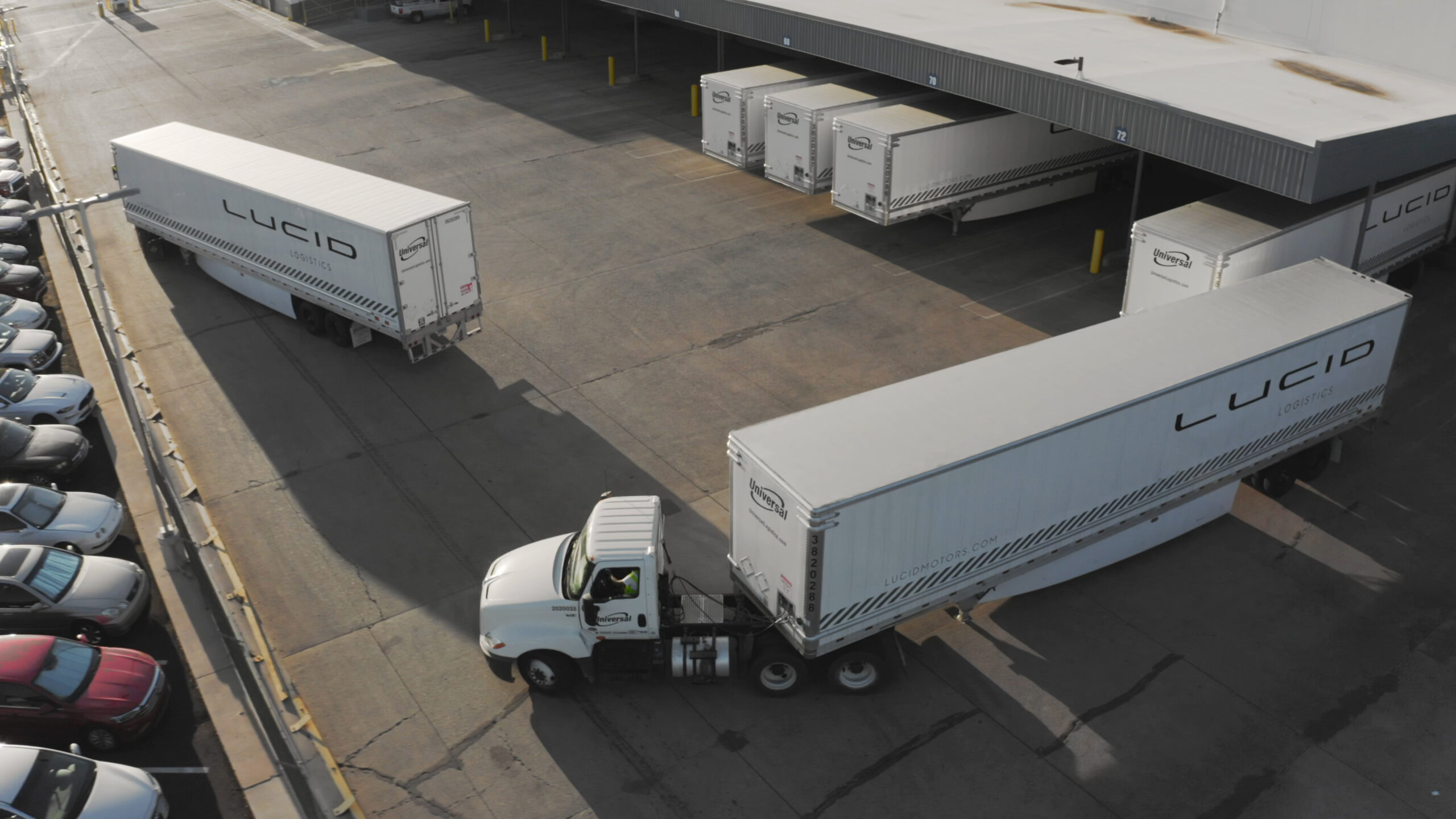## Lucid Motors Lot With Lucid Airs – September 7th Edition

September 7, 2022Bear’s Workshop on YouTube did a flyover of the Lucid Motors factory plant today September 7, 2022, in Casa Grande, Arizona.

I am not posting any comments or commentary.

Remember, there are some theories on what the various sections of the lot mean.

Here are the photos from the flyovers of the Lucid Motors production factory:

Here is the September 7, 2022 video where the screenshot was taken:

Here is the September 3, 2022 video where the screenshot was taken:

Here is the August 31, 2022 video where the screenshot was taken:

Here is the August 27, 2022 video where the screenshot was taken:

Here is the August 23, 2022 video where the screenshot was taken:

Here is the August 19, 2022 video where the screenshot was taken:

Here is the August 16, 2022 video where the screenshot was taken:

Here is the August 10, 2022 video where the screenshot was taken:

And maybe a stealth prototype?

Here is the August 8, 2022 video where the screenshot was taken:

Here is the August 2, 2022 video where the screenshot was taken:

Here is the July 28, 2022 video where the screenshot was taken:

Here is the July 26, 2022 video where the screenshot was taken:

Here is the July 23, 2022 video where the screenshot was taken:

Here is the July 21, 2022 video where the screenshot was taken:

Here is the July 19, 2022 video where the screenshot was taken:

Here is the July 15, 2022 video where the screenshot was taken:

Here is the July 11, 2022 video where the screenshot was taken:

Wide low-resolution version:

Here is the July 7, 2022 video where the screenshot was taken:

Here is a low resolution but wider view:

Here is the July 5, 2022 video where the screenshot was taken:

Here is the June 30, 2022 video where the screenshot was taken:

Here is the June 28, 2022 video where the screenshot was taken:

Here is the June 23, 2022 video where the screenshot was taken:

Here is the June 20, 2022 video where the screenshot was taken:

Here is the June 15, 2022 video where the screenshot was taken:

Here is the June 13, 2022 video where the screenshot was taken:

Here are zoom ins of those metal roof Lucid Airs:

Here is the June 8, 2022 video where the screenshot was taken:

Here is the June 6, 2022 video where the screenshot was taken:

Here is the June 3, 2022 video where the screenshot was taken:

Here is the June 1, 2022 video where the screenshot was taken:

Here is the May 25, 2022 video where the screenshot was taken:

Here is the May 23, 2022 video where the screenshot was taken:

Here is the May 18, 2022 video where the screenshot was taken:

Here is the May 16, 2022 video where the screenshot was taken:

Here is the May 11, 2022 video where the screenshot was taken:

Here is the May 9, 2022 video where the screenshot was taken:

Here is the May 5, 2022 video where the screenshot was taken:

Here is the May 2, 2022 video where this screenshot was taken:

Here is a wider shot showing the top lot as well with some cars in it:

Here is the photo from the April 29th video over here:

Compare that to the April 25th version over here:

Here is the April 20th shot of the lot from this video:

Here is zoomed in on the lower lot:

Here is the April 18th shot of the lot from this position in this video:

Here is the April 13th shot of the lot from this position in this video:

Here is the April 11th shot of the lot from this position in this video:

Compared to the April 4th thot of the lot from this position in this video: